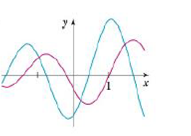Chapter 2.8, Problem 48E### Single Variable Calculus: Early Tr...

8th Edition
James Stewart
ISBN: 9781305270343

#### Solutions

Chapter
Section### Single Variable Calculus: Early Tr...

8th Edition
James Stewart
ISBN: 9781305270343
Textbook Problem

# The graphs of a function f and its derivative f' are shown. Which is bigger, f'( –1) or f"(l)?To determine

To Identify: The bigger quantity among f(1) and f(1) with proper explanation.

Explanation

Note:

The derivative is zero where the function has a horizontal tangent.

Graph:

The given graph of f and f is shown in Figure 1.

Observation:

From Figure 1 it is observed that the point where curve c2 has functional value 0 is the same point where graph of curve c1 has horizontal tangent.

By note, c2 is the derivative of the graph curve c1.

Thus, c1=f and c2=f.

Calculation:

From Figure 1, f(1)>0 as the graph of f lies above the x-axis for x=1.

Thus, f(1)>0 (1)

From Figure 1, f(x) has horizontal tangent at x=1.

By note, the value of the derivative of f at x=1 is 0

### Still sussing out bartleby?

Check out a sample textbook solution.

See a sample solution

#### The Solution to Your Study Problems

Bartleby provides explanations to thousands of textbook problems written by our experts, many with advanced degrees!

Get Started

#### In Exercises 99-106, factor out the greatest common factor from each expression. 99. 4x5 2x4 6x3

Applied Calculus for the Managerial, Life, and Social Sciences: A Brief Approach

#### Factor each expression in Problems 7-18 as a product of binomials. 13.

Mathematical Applications for the Management, Life, and Social Sciences

#### The solution to y = y2 with y(1)=13 is: a) y=1x+2 b) y=lnx+13 c) y=12x216 d) y=12x16

Study Guide for Stewart's Single Variable Calculus: Early Transcendentals, 8th

#### True or False: div curl F = 0.

Study Guide for Stewart's Multivariable Calculus, 8th